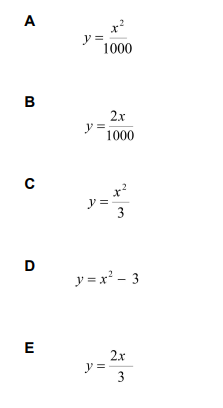# IMAT 2015 Q53 [Expression]

Given that 2 log_{10} (x) – 3 = log_{10} (y)

Express y in terms of x.In order to solve this, we need to know some rules of the logarithm:

1. \log _{a}x\cdot y = \log _{a}x + \log _{a}y
2. \log _{a}\frac{x}{y} = \log _{a}x - \log _{a}y
3. \log _{a} x^{m} = m \log _{a}x
4. \log _{a} \sqrt[n]{x} = \frac{1}{n} \log _{a}x
5. \log _{a}1 = 0
6. \log _{a}a= 1
7. \log _{a}a^{k} = k
8. a^{\log _{a}k} = k

There are multiple ways to solve 2\log _{10}(x) -3 = \log _{10}(y).

I will solve it in one way that I think is the fastest (using rule 3 and 8):

2\log _{10}(x) -3 = \log _{10}(y) \to (rule 3)\log _{10}(x^2) -3 = \log _{10}(y)
\to (using 10^{function}) 10^{\log _{10}(x^2) -3} = 10^{\log _{10}(y)} \to 10^{\log _{10}(x^2)} \cdot 10^{-3}= 10^{\log _{10}(y)}
\to (rule 8) (x^2)\cdot 10^{-3} = (y) \to y = \frac{x^2}{10^3} \to y= \frac{x^2}{1000}

Which gives us the correct answer (A)